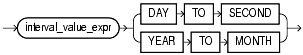## INTERVAL Expressions

An interval expression yields a value of `INTERVAL` `YEAR` `TO` `MONTH` or `INTERVAL` `DAY` `TO` `SECOND`.

interval_expression::=Text description of interval_expression

The `interval_value_expr` can be the value of an `INTERVAL` column or a compound expression that yields an interval value. Datetimes and intervals can be combined according to the rules defined in Table 2-5. The six combinations that yield interval values are valid in an interval expression.

For example, the following statement subtracts the value of the `order_date` column in the sample table `orders` (a datetime value) from the system timestamp (another datetime value) to yield an interval value expression:

```SELECT (SYSTIMESTAMP - order_date) DAY TO SECOND from orders;
```